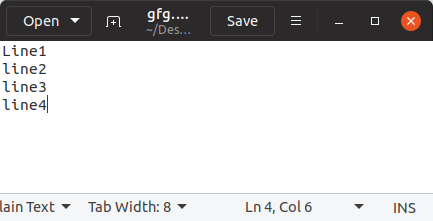# Count number of lines in a text file in Python

• Difficulty Level : Easy
• Last Updated : 08 Jul, 2022

Prerequisites: File Handling in Python

Counting the number of characters is important because almost all the text boxes that rely on user input have a certain limit on the number of characters that can be inserted. For example, If the file is small, you can use readlines() or an A loop approach. If you have a lot of files to work with To determine line count, use the Generator and Raw interface,  If you don’t want to load the complete file in, utilize a loop and enumerate() for huge files. This program in Data file handling in Python emphasizes counting the number of lines in a text file in Python

Below is the implementation.

Let’s suppose the text file looks like this –myfile.txt

### Method 1: Python count numbers of lines in a string using readlines()

The readlines() are used to read all the lines at a single go and then return them as each line a string element in a list. This function can be used for small files

## Python3

 `with ``open``(r``"myfile.txt"``, ``'r'``) as fp:` `    ``lines ``=` `len``(fp.readlines())` `    ``print``(``'Total Number of lines:'``, lines)`

Output:

`Total Number of lines: 5`

### Method 2: Python count number of lines in text file using enumerate

Enumerate() method adds a counter to an iterable and returns it in a form of enumerating object.

## Python3

 `with ``open``(r``"myfile.txt"``, ``'r'``) as fp:` `    ``for` `count, line ``in` `enumerate``(fp):` `        ``pass` `print``(``'Total Number of lines:'``, count ``+` `1``)`

Output:

`Total Number of lines: 5`

### Method 3: Use Loop and Counter to Count Lines

Loops python is used for sequential traversal. here we will count the number of lines using the loop and if statement. if help us to determine is there any character or not, if there will be any character present the IF condition returns true and the counter will increment.

## Python3

 `# Opening a file` `file` `=` `open``(``"gfg.txt"``, ``"r"``)` `Counter ``=` `0`   `# Reading from file` `Content ``=` `file``.read()` `CoList ``=` `Content.split(``"\n"``)`   `for` `i ``in` `CoList:` `    ``if` `i:` `        ``Counter ``+``=` `1`   `print``(``"This is the number of lines in the file"``)` `print``(Counter)`

Output:

```This is the number of lines in the file
4```

### Method 4: Use Loop and Sum function to Count Lines

Loops python is used for sequential traversal. here we will count the number of lines using the sum function in Python. if help us to determine is there any character or not, if there will be any character present the IF condition returns true and the counter will increment.

## Python3

 `with ``open``(r``"myfile.txt"``, ``'r'``) as fp:` `    ``lines ``=` `sum``(``1` `for` `line ``in` `fp)` `    ``print``(``'Total Number of lines:'``, lines)`

Output:

`Total Number of lines: 5`

My Personal Notes arrow_drop_up
Recommended Articles
Page :